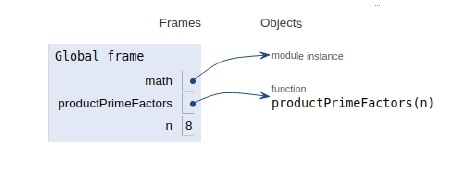# Python Program for Product of unique prime factors of a number

Problem statement − Given a number n, we need to find the product of all of its unique prime factors available and return it.

For example,

Input: num = 11
Output: Product is 11
Explanation:
Here, the input number is 11 having only 1 prime factor and it is 11.
And hence their product is 11.

## Approach 1

Using a for loop from i = 2 to n+1 check whether i is a factor of n & then check if i is the prime number itself, if yes then store product in product variable and continue this process until I become = n.

#### Example

Live Demo

def productPrimeFactors(n):
product = 1
for i in range(2, n+1):
if (n % i == 0):
isPrime = 1
for j in range(2, int(i/2 + 1)):
if (i % j == 0):
isPrime = 0
break
if (isPrime):
product = product * i
return product
# main
n = 18
print (productPrimeFactors(n))

#### Output

6

The scope of all the variables are shown in the image below −## Approach 2

• While n is divisible by 2(even), print 2 and divide n by 2.

• After step 1, n must become odd. Now start a for loop from i = 3 till the square root of n. While I divides n, print I and divide n by i. After I fail to divide n, increment I by 2 and continue the process.

• If n is a prime number and is greater than 2, then n will not become 1 by above two steps. Hence print n if it is greater than 2.

#### Example

import math
def productPrimeFactors(n):
product = 1
# prime factor 2
if (n % 2 == 0):
product *= 2
while (n%2 == 0):
n = n/2
# n must be odd
for i in range (3, int(math.sqrt(n)), 2):
# While i divides n, print i and
# divide n
if (n % i == 0):
product = product * i
while (n%i == 0):
n = n/i
# n is a prime number greater than 2
if (n > 2):
product = product * n
return product
# main()
n = 8
print (int(productPrimeFactors(n)))

#### Output

2

The scopes of the variables are mentioned in the image below −## Conclusion

In this article, we learned about the product of unique prime factors of a given number with a brute force approach and an efficient approach.

Updated on: 11-Sep-2019

826 Views